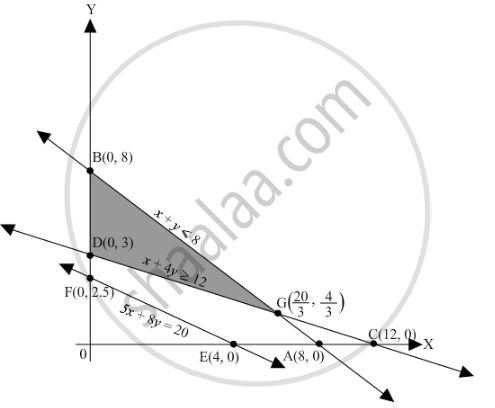# Minimize Z = 30x + 20y Subject to X + Y ≤ 8 X + 4 Y ≥ 12 5 X + 8 Y = 20 X , Y ≥ 0 - Mathematics

Minimize Z = 30x + 20y
Subject to

$x + y \leq 8$
$x + 4y \geq 12$
$5x + 8y = 20$
$x, y \geq 0$

#### Solution

First, we will convert the given inequations into equations, we obtain the following equations:
x + y = 8, x + 4y = 12, x = 0 and y = 0

5x + 8y = 20 is already an equation.

Region represented by x + y ≤ 8:
The line x + y = 8 meets the coordinate axes at A(8, 0) and B(0, 8) respectively. By joining these points we obtain the line x + y = 8.Clearly (0,0) satisfies the inequation xy ≤ 8. So,the region in xy plane which contain the origin represents the solution set of the inequation x + y ≤ 8.

Region represented by x + 4y ≥ 12:
The line x + 4y = 12 meets the coordinate axes at C(12, 0) and D(0, 3) respectively. By joining these points we obtain the line x + 4y = 12.Clearly (0,0) satisfies the inequation x + 4y ≥ 12. So,the region in xy plane which does not contain the origin represents the solution set of the inequation x + 4y ≥ 12.

The line 5x + 8y = 20 is the line that passes through E(4, 0) and $F\left( 0, \frac{5}{2} \right)$ Region represented by x ≥ 0 and y ≥ 0:
Since, every point in the first quadrant satisfies these inequations. So, the first quadrant is the region represented by the inequations x ≥ 0 and ≥ 0.

The feasible region determined by the system of constraints, x + y ≤ 8, x + 4y ≥ 12, 5x+ 8y = 20, x ≥ 0 and y ≥ 0 are as follows.The corner points of the feasible region are B(0,8), D(0,3),

$G\left( \frac{20}{3}, \frac{4}{3} \right)$

The values of Z at these corner points are as follows.

 Corner point Z = 30x + 20y B(0,8) 160 D(0,3) 60 $G\left( \frac{20}{3}, \frac{4}{3} \right)$ 266.66

Therefore, the minimum value of Z is 60 at the point D(0,3).  Hence, x = 0 and y =3 is the optimal solution of the given LPP.
Thus, the optimal value of Z is 60

Concept: Graphical Method of Solving Linear Programming Problems
Is there an error in this question or solution?

#### APPEARS IN

RD Sharma Class 12 Maths
Chapter 30 Linear programming
Exercise 30.2 | Q 12 | Page 32